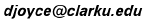# Continued Fractions

Continued fractions are a way of approximating real numbers by nearby rational numbers. The more terms there are in the continued fraction, the closer the rational number will be to the given real number.

Let x be the given real number. The first step in finding the continued fraction for x is to find the largest integer a less than or equal to x. Sometimes this is called the "greatest integer" in x and sometimes it's called the "floor" of x. We'll call it the floor. For example, the floor of 7/3 is 2. Note that the floor of an integer is that integer itself. For example, the floor of 3 is 3. In the following diagram, all numbers between 0 and 1 have a floor value of a = 0; those between 1 and 2 have a floor value of 1, indicated in light red; those between 2 and 3 have a floor value of 2, indicated in orange; and so forth.

(If you can read these words, you're only seeing a captured image, not the real java applet! You need a browser that deals with java to move the points.)Now that the integer part a of the real number x is taken care of, the remainder x – a is a number between 0 and 1. The next step is to take the reciprocal of that number

y = 1/(a – x)

and do the same thing to it. Start by taking its floor b. Since y is close to b, therefore 1/b is close to y which is x – a. Therefore, a + 1/b is close to x.

The next diagram shows the value for b on the second line below the values for a. The same color coding is used.

(If you can read these words, you're only seeing a captured image, not the real java applet! You need a browser that deals with java to move the points.)These figures utilize the Geometry Applet.

April, 2006.
David E. Joyce
Department of Mathematics and Computer Science
Clark University
Worcester, MA 01610The address of this file is http://aleph0.clarku.edu/~djoyce/java/Geometry/contfract.html